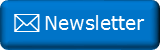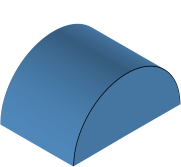﻿ Centroids of semicircles and hemispheresNewsletter: Click here to join an email list and be contacted when a new article is published.      Advertisement

# Centroids of semicircles and hemispheresWhere is the centroid of a semicircle? If you cut out a semicircle from a sheet of uniform density cardboard, over which point could you, carefully, balance it on the top of a pin? If you don’t know the answer, think about it for a couple of seconds. Does you intuition give you a clue?

### Solution

Let’s use some calculus to derive the answer.:We can model a semicircle as a stack of infinitesimally thin strips. If we sum the moment of all these strips, normalised over the area of the entire half-circle, we can find the centroid. The centroid is the location that we can model the shape as if the entire weight acts through this point.

From symmetry, we can see that the centroid lies along the y-axis, which acts like a mirror to the quarter circles either side. We just need to determine how far up this point is.This is approximately 42.44% of the radius.

I don't know about you, but this answer surprised me a little. I would not have predicted it would be an irrational percentage.

### Moving to 3DIf a semicircle is extruded into an arch (of uniform density), then the centroid stays at the 42.44% position from the base. But where is the centroid of a hemi-sphere? Is it the same? Let's take a look …

### HemisphereDo you think this shape will have a different centroid? As before we can model this as a pile of infinitesimally thin circular pancakes stacked ontop of each other. We can sum up the moment of all these disks and divide by the volume of half the sphere. Do you have any intuition about the result?This is 37.50% of the radius.

This is an interesting result. It's a little cleaner than the formula for a semicircle and does not involve π. The centroid is a little nearer the base of the dome.

Is this a result you expected? It makes sense when you consider that a hemisphere tapers in two axes as it moves from base to tip, so we should expect the centroid to be nearer the base.

You can find a complete list of all the articles here.Click here to receive email alerts on new articles.